# 349. Circumcircle Investigation

The A-level textbook we use has a nice picture of the circumcircle of a triangle and a definition, plus a brief description of how to work through them. For those who are pondering what a circumcircle is, click on the image or link below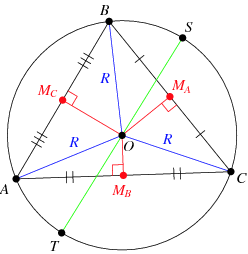Image credit: WolframMathWorld

I’ll just stick to basic vocabulary in this post, rather than the formal circumcentre and circumradius.

Back to the book – not exactly inspiring or memorable stuff!

I looked at the class and off the cuff changed the lesson plan.

Equipment

• Plain paper
• Pencil
• Ruler
• Compasses
• Calculator

Step 1

Draw a decent size triangle on the paper. Label the corners A,B,C.

Step 2

Using geometrical constructions, find the centre of the circle that your triangle fits in. Check by actually drawing the circle

Step 3

Discuss what techniques gave the best results – hopefully you’ll have perpendicular bisectors. There is a nice comparison between bisecting the angles (which some students will do) and bisecting the sides. The angle bisectors always cross inside the triangle, the side bisectors don’t.

Step 4

Randomly generate co-ordinates for A, B, & C. Get the students to pick them and then they can’t moan if the calculations are awful.

Step 5

Discuss how you are going to find the centre and radius of the circumcircle. We decided on:

• Only use two sides
• Find the midpoints
• Find the gradients and hence perpendicular gradients
• Generate the equations of the lines through the midpoint
• Find where they intersect
• Use the point and one corner to find the radius

Step 6

Review their methods, looking for premature rounding in questions. I’m still instilling an appreciation for the accuracy of fractions and surds, over reaching for the calculator.

Step 7

This is how my solution looked – I numbered the picture and the steps so students could follow the logic. I was answering on one page projected on screen.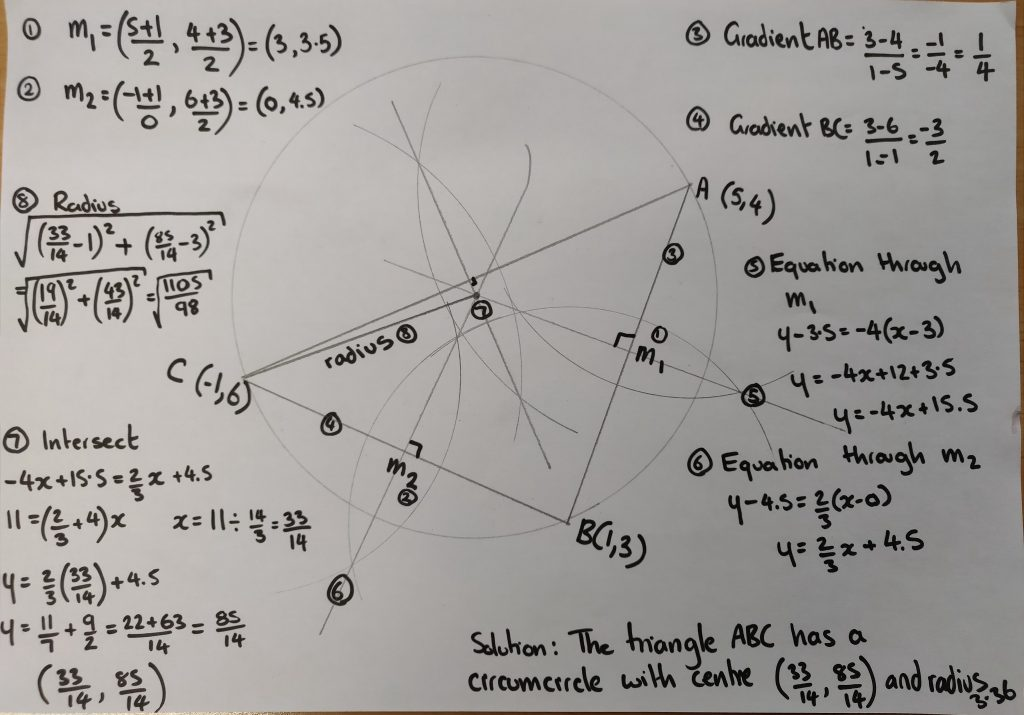# 250. Crack the Circle

Here’s a quick resource for consolidating and revising the area and perimeter of circles and semi-circles.

Pupils complete the worksheet then work out the code. I personally like having a clear glass Kilner-style jar with a combination padlock at the front of the class … with a little treat for the class to aim for securely locked inside!

# 245. Fair share

I spotted this ‘Expert Tip’ whilst flicking through a supermarket magazine: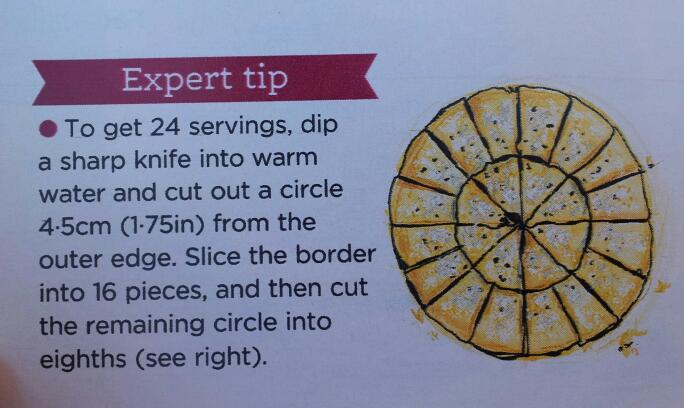Image credit: tesco.com/foodandliving

Question
If this cake has a diameter of 18cm (7in), is this a fair way to split it between guests? Can you prove your result in general terms?

Of course, this assumes that the icing on the side doesn’t count in the diameter or guest preference.

# 233. Stealthy Cone Investigation

I like to encourage students to discover rules and formulae for themselves. It’s important that students understand where the maths comes from so they can apply their skills effectively. They also don’t have to rely on remembering a rule (which they may forget when they are stressed).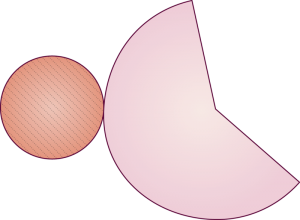Image credit: http://www.ck12.org/geometry/Surface-Area-and-Volume-of-Cones/

This resource is a neat and effective way to investigate the surface area of a cone through measuring circles and creating a 3D shape. Students get a physical feel for how the dimensions fit together. Throughout the lesson I let students choose their degree of accuracy in cutting, measuring and calculating. Of course, when we discussed the ‘solution’ at the end of the session it was impossible for me to put one correct answer on the board. So I generalised using a and b for the radii – explaining that everyone could check their method in general terms. The lovely ‘penny drop’ moment happened when my a’s and b’s suddenly became a general rule. I’d conned the class into using algebra because of the accuracy issue.

Download the worksheet and answers here: Surface_area_of_a_cone

Hint: Copying onto coloured paper or card makes this activity stand out in their notes.

# 216. Back to the Takeaway

If you like Takeaway homeworks or need a resource for the area and perimeter of circles, including some arc/sector challenges, then my third takeaway homework is for you!

Takeaway Homework 3: Area & circumference of circles

# 187. Clever circles

Here is a quick, multi-function resource for you: a set of overlapping circles for angles, pie-charts and fractions/percentages.

Equipment
Card
Scissors
Pencil
Straight edge or ruler
Pair of compasses
A 360 degree protractor printed on paper (or a tracing paper protractor cut out)

Construction
1. Cut out three identical circles and the paper protractor.

2. Stack them on top of each other and put the pointy end of the compasses (or a drawing pin) through the middle. Wiggle it around to make a bigger hole – please don’t stab yourself.

3. Draw a radius on the circles.

4. Cut down each radius on the circles and the 0 degree line on the protractor.
5. All done!

Activity 1: Angle Estimation
Slot two circles together:

Estimate the orange angle.
What could the blue angle be?
Show me an acute angle.
Show me a blue 170 degree angle.

Activity 2: Reading a protractor scale
Slot the protractor into a circle:

How big is the blue angle?
Show me an 80 degree angle.

Activity 3: Pie-charts
Slot the three circles together:

What could this pie-chart represent?
Show me a pie chart with two equal sections

Activity 4: Fractions and percentages
Use three circles again:

Estimate what percentage is purple.
What fraction could the blue section represent?

# 154. When will I need to work out the area of a circle?

Answer:
If this is your crop on your farm!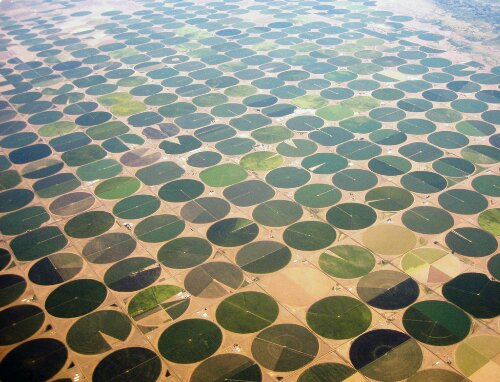This is center pivot (or central pivot or circle) irrigation. The area which is watered is pretty obvious. There are plenty of images on the internet and sites full of statistics.

I could imagine this making a good research homework. Apart from simply working out the fertile area, you could look at volume of water used – you could even work out the optimum size and number of circles for maximum coverage!